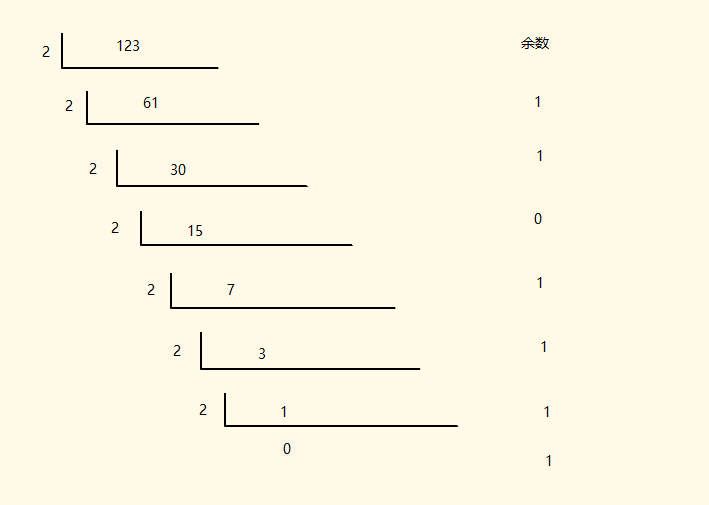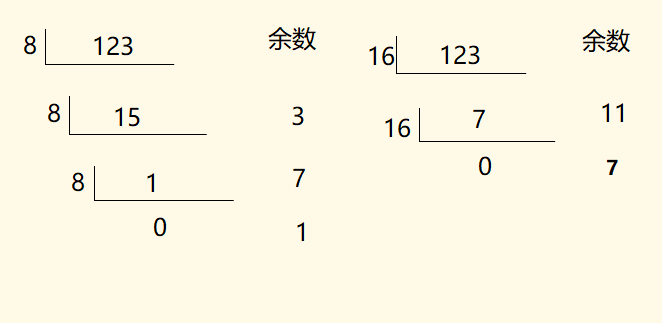# java常用的进制之间转换

int a = 18;

int a = 18;

int a = 123;<p>余数倒叙排列 1111011</p><p>十进制转成对应八进制,余数倒叙排列 173  </p>
<p>十进制转成对应十六进制,余数倒叙排列 7b  </p>
<p>兄弟们也可以用函数去验证一下 :  </p>
<p>Integer.toBinaryString(123)</p>
<p>Integer.toOctalString(123)</p>
<p>Integer.toHexString(123)</p>
<p>顺带一句</p>
<p>也可以取字符的二进制,Integer.toBinaryString('A'); 字符 A 对应的ascoll码值 65的二进制 1000001</p>

二进制,八进制,十六进制 转成 十进制

int a = 0b10010;
//你可以把10010,放在数组里面,[1,0,0,1,0]

0   0
1   1
0   2
0   3
1   4

int b = 022;
2*8^1+2*8^0 = 18

int c = 0X12;
1*16^1+2*16^0 = 18

Integer.parseInt("10010",2)
Integer.parseInt("22",8)
Integer.parseInt("12",16)

int a = 0b10010;
10010,前面补 0,得 010010,
010  010
2    2 => 最终结果为 22

int a = 0b10010;
10010,前面补 0,得 00010010,
0001  0010
1    2 =>最终结果为 12

### 十进制小数与二进制之间的相互转换

int a = 0.125;
0.125*2 = 0.25 整数部分 0
0.25*2 = 0.5   整数部分 0
0.5*2 = 1      整数部分 1

0*2^-1 + 0*2^-2 + 1*2^-3 = 0.125
负数怎么转换呢?

int a = 5; // 正数

int b = -5; // 负数

10-0810-243841
05-26293
02-214万+
06-093万+